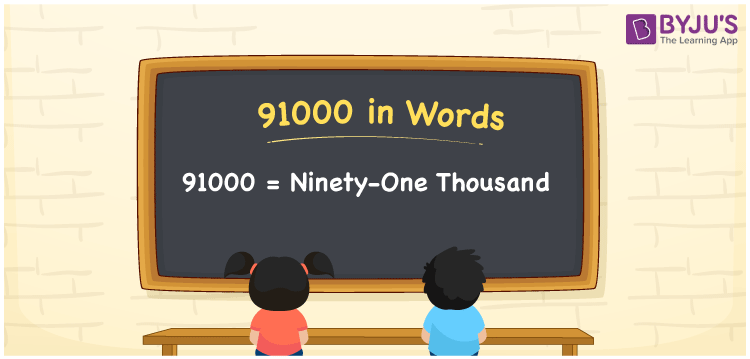# 91000 in Words

We can write 91000 in words as Ninety-one thousand. Suppose your annual house rent is Rs. 91000, and this can be conveyed as, “My annual house rent is Ninety-one thousand rupees”. However, we can use the place value chart to define the number 91000 in words. Let’s learn how to write the cardinal number 91000 in words, along with some interesting facts about 91000.

 91000 in words Ninety-one thousand Ninety-one thousand in Numbers 91000

## 91000 in English Words

Using the English alphabet, we generally write numbers in words. Thus, we can spell 91000 in English as “Ninety-one thousand”.## How to Write 91000 in Words?

The number 91000 has five digits so we need to make a place value chart with five columns as shown below.

 Ten thousands Thousands Hundreds Tens Ones 9 1 0 0 0

Here, ones = 0, tens = 0, hundreds = 0, thousands = 1, ten thousands = 9

These digits can be expanded as:

9 × Ten thousand + 1 × Thousand + 0 × Hundred + 0 × Ten + 0 × One

= 9 × 10000 + 1 × 1000 + 0 × 100 + 0 × 10 + 0 × 1

= 90000 + 1000

= Ninety thousand + One thousand

= Ninety-one thousand

Therefore, 91000 in words is written as Ninety-one thousand.

91000 is a natural number that precedes 91001 and succeeds 90999.

91000 in words – Ninety-one thousand

Is 91000 an odd number? – No

Is 91000 an even number? – Yes

Is 91000 a perfect square number? – No

Is 91000 a perfect cube number? – No

Is 91000 a prime number? – No

Is 91000 a composite number? – Yes

## Frequently Asked Questions on 91000 in Words

Q1

### How do you write 91000 in words?

The number 91000 can be written in English words as Ninety-one thousand.
Q2

### How do you write 91000 rupees in words on a cheque?

On a cheque, we generally write 91000 rupees in words as “Ninety-one thousand rupees only”.
Q3

### Express the value of 45000 + 46000 in words.

45000 + 46000 = 91000 Therefore, the value of 45000 + 46000, i.e. 91000 can be expressed in words as Ninety-one thousand.